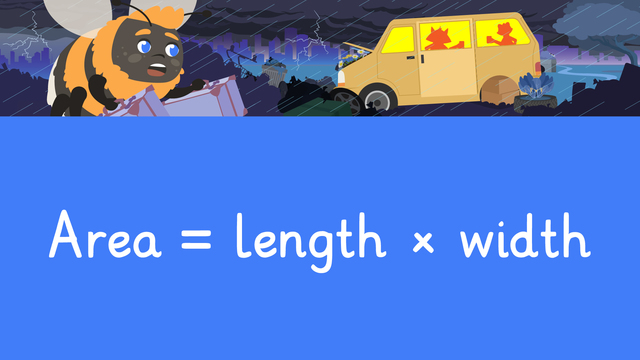# Area in Square CentimetersRating

Ø 5.0 / 1 ratings

The authorsTeam Digital
Area in Square Centimeters
CCSS.MATH.CONTENT.4.MD.A.3

## Basics on the topicArea in Square Centimeters

### Content

#### How do you Find Area in Square Centimeters?

We find the area of a rectangular shape by using the formula, a = l x w#### In this Video

Zuri and Freddie have an unwanted house guest and come up with a plan to get him to leave. We learn how to find the area in square centimeters to help build a perfect home for Mr. Bee.

#### Area in Square Centimeter ExampleLabel the area in square centimeters.#### Follow-Up Activities on How to Find the Area

After the video, there are additional exercises and worksheets that give continued practice on how to find area.

### TranscriptArea in Square Centimeters

[Nervous because he doesn’t want to get stung] “Zuri, what are we going to do?” “I don’t think he plans on going anywhere.” “Maybe we can convince him to leave by giving him a nice place of his own?” “Yes, we can build him the perfect place to live!” We can help Zuri and Freddie build their unwanted guest the perfect home by measuring ... “Area in Square Centimeters”. When measuring shapes, we can find the distance around the outside of the shape, called the perimeter... and the amount of space a shape covers on the inside. AREA is the measurement of the INSIDE of a shape. It is the amount of space taken up by an object on a flat surface. We can find the area of a rectangular shape by using the formula, Area equals length times width... or equals times for short. Using this formula and the two measurements we do know, we can solve for unknown parts. Zuri and Freddie found some popsicle sticks in the landfill and decided to make Mr. Bee a lovely fenced-in flower garden! The garden covers ninety-six square centimeters in all... and the garden is eight centimeters wide. The measurement we are missing is the length of the garden. In order to solve, we put all the numbers into the formula... and read this as ninety-six equals the length times eight. To solve for the length, we ask ourselves, what number times eight is equal to ninety-six? (...) Twelve times eight equals ninety-six... so the length is twelve centimeters. Zuri and Freddie found some building blocks and thought these would make a great front porch! The area of the porch measures two hundred fifty square centimeters in all… and the length is twenty-five centimeters. What is the missing measurement? (...) We need to solve for the width. First, we replace the formula with the numbers we know. The equation is two- hundred -fifty equals twenty-five times . To solve for the missing width, we want to ask ourselves (...) 'twenty-five times what number equals two-hundred-fifty?'. What is the width? (...) The width of the porch is ten centimeters. Zuri and Freddie found the PERFECT house...an old box! Look at the dimensions of the area covered in this space. What do we know about the area of the box? (...) We know that the total area covered is seven hundred square centimeters… and the length is thirty-five centimeters. What is the equation for the area of the box? (...) It is seven hundred equals thirty-five times the width. How do we solve the equation? (...) We ask ourselves, 'thirty-five times what number equals seven hundred?' This time, try to find the missing measurement on your own. Pause the video so you have time to work (...) and press play again when you're ready to see the answer! What is the width of the box? It is twenty centimeters since thirty-five times twenty is seven hundred. In the comment section, describe the way you solved this problem. (...) Remember (...) Area is the measurement of the INSIDE of a shape. We solve for the area of a rectangular shape by using the formula, equals times . Replace the measurements in the equation with what we know... and use multiplication strategies to solve for the missing information. "Gee Zuri,(...) I sure am going to miss him!"